Estimating Terminal Value

Since you cannot estimate cash flows forever, you generally impose closure in discounted cash flow valuation by stopping your estimation of cash flows sometime in the future and then computing a terminal value that reflects the value of the firm at that point.

Value of a Firm =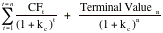You can find the terminal value in one of three ways. One is to assume a liquidation of the firm’s assets in the terminal year and estimate what others would pay for the assets that the firm has accumulated at that point. The other two approaches value the firm as a going concern at the time of the terminal value estimation. One applies a multiple to earnings, revenues or book value to estimate the value in the terminal year. The other assumes that the cash flows of the firm will grow at a constant rate forever – a stable growth rate. With stable growth, the terminal value can be estimated using a perpetual growth model.

## Liquidation Value

In some valuations, we can assume that the firm will cease operations at a point in time in the future and sell the assets it has accumulated to the highest bidders. The estimate that emerges is called a liquidation value. There are two ways in which the liquidation value can be estimated. One is to base it on the book value of the assets, adjusted for any inflation during the period. Thus, if the book value of assets ten years from now is expected to be \$2 billion, the average age of the assets at that point is 5 years and the expected inflation rate is 3%, the expected liquidation value can be estimated.

Expected Liquidation value = Book Value of AssetsTerm yr (1+ inflation rate)Average life of assets

= \$ 2 billion (1.03)5 = \$2.319 billion

The limitation of this approach is that it is based upon accounting book value and does not reflect the earning power of the assets.

The alternative approach is to estimate the value based upon the earning power of the assets. To make this estimate, we would first have to estimate the expected cash flows from the assets and then discount these cash flows back to the present, using an appropriate discount rate. In the example above, for instance, if we assumed that the assets in question could be expected to generate \$400 million in after-tax cash flows for 15 years (after the terminal year) and the cost of capital was 10%, your estimate of the expected liquidation value would be:

Expected Liquidation value =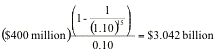When valuing equity, there is one additional step that needs to be taken. The estimated value of debt outstanding in the terminal year has to be subtracted from the liquidation value to arrive at the liquidation proceeds for equity investors.

## Multiple Approach

In this approach, the value of a firm in a future year is estimated by applying a multiple to the firm’s earnings or revenues in that year. For instance, a firm with expected revenues of \$6 billion ten years from now will have an estimated terminal value in that year of \$12 billion if a value to sales multiple of 2 is used. If valuing equity, we use equity multiples such as price earnings ratios to arrive at the terminal value.

While this approach has the virtue of simplicity, the multiple has a huge effect on the final value and where it is obtained can be critical. If, as is common, the multiple is estimated by looking at how comparable firms in the business today are priced by the market. The valuation becomes a relative valuation rather than a discounted cash flow valuation. If the multiple is estimated using fundamentals, it converges on the stable growth model that will be described in the next section.

All in all, using multiples to estimate terminal value, when those multiples are estimated from comparable firms, results in a dangerous mix of relative and discounted cash flow valuation. While there are advantages to relative valuation, and we will consider these in a later chapter, a discounted cash flow valuation should provide you with an estimate of intrinsic value, not relative value. Consequently, the only consistent way of estimating terminal value in a discounted cash flow model is to use either a liquidation value or a stable growth model.

## Stable Growth Model

In the liquidation value approach, we are assuming that your firm has a finite life and that it will be liquidated at the end of that life. Firms, however, can reinvest some of their cash flows back into new assets and extend their lives. If we assume that cash flows, beyond the terminal year, will grow at a constant rate forever, the terminal value can be estimated as.

Terminal Valuet =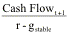where the cash flow and the discount rate used will depend upon whether you are valuing the firm or valuing the equity. If we are valuing the equity, the terminal value of equity can be written as:

Terminal value of Equityn =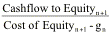The cashflow to equity can be defined strictly as dividends (in the dividend discount model) or as free cashflow to equity. If valuing a firm, the terminal value can be written as:

Terminal valuen =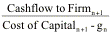where the cost of capital and the growth rate in the model are sustainable forever.

In this section, we will begin by considering how high a stable growth rate can be, how to best estimate when your firm will be a stable growth firm and what inputs need to be adjusted as a firm approaches stable growth.